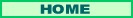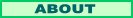//-->µ Machine Understanding

Search

More Tech Stuff:

Indexing Books: Lessons in Language Computations

Client-Side Frame Manipulation Inside the Microsoft Internet Explorer Object Model with Visual Basic .NET

Replacing a PC power supply

Constructing a Mandelbrot Set Based Logo with Visual Basic.NET and Fireworks

June 10, 2010

Understanding Probability and Probabilistic Reasoning

I think I mentioned earlier that I have had problems with the Numenta model as described in "Towards a Mathematical Theory of Cortical Micro-circuits" because of the use of probability-based mathematics. It seems to me that neurons are deterministic mechanisms. But I have noted in the past that I can be pretty dim-witted at times, and decided to study Numenta's HTM systems anyway. I reminded myself that quantum physics has two different formulations, one based on matrix algebra and the other on the Schrodinger equation. They both work, and some brilliant person showed that they are formally the same long, long ago. So when thinking about or solving problems you can use whichever is easiest or gives the best insights. The same way some physics and math problems are easier in polar coordinates than in rectangular coordinates.

Months ago I ground to a halt in my reading of Judea Pearl's Probabilistic Reasoning in Intelligent Systems, which provides much of the background to the Numenta discussion. Yesterday I decided to tackle it again and commenced reading at page 143. I noticed that some notation was ambiguous, which is typical of expert writers who assume their readers are right up with them. So I decided to go back and make sure that P(A,B) really does mean the probability that both A and B are true. I thought I'd make sure I understood the Bayes interpretation of probability as well.

I ended up reading starting at page 29, Chapter 2, Bayesian Inference, 2.1, Basic Concepts, 2.1.1 Probabilistic Formulation and Bayesian Inversion. Note that I took two semesters of logic and one semester of probability in college, and as part of my profession deal with biostatistics, the kind reported from clinical trials, on a regular basis. Note also that I have studied philosphic issues of quantum physics and even the math involved.

Yet when I read this simple introduction this time, the scales fell from my eyes, or from my cortical networks.

With probabilistic reasoning, it is fair to say that we are not talking about rolling dice (even though Pearl uses the familar probabilities of two-die rolls to illustrate some points).

We are talking about the math of pobability theory. For most practical purposes, that is the math of fractions. Third or fourth grade stuff. (I had a fifth grade teacher I hated, Mrs. Lopez, who was all about memorizing things. We memorized the decimal equivalents of about 50 common fractions. I knew I could always get the decimal equivalent by dividing, so considered this a stupid exercise. )

When thinking about human memory, you can safely substitute "percentage of like situations" for probability.

Updating the "percentage of like situations" based on experience makes sense. Since we can test for novel situations, like "both A and B" or "A and not C, given B", by multiplying, adding, or subtracting fractions, these updates may effect a chain of knowledge or deductions across the brain (or mind, if you prefer).

Calling all background information and assumptions a person has K (I don't know why K, maybe it stands for Knowledge), I quote Pearl page 30: "However, when the background information undergoes changes, we need to identify specifically the assumptions that account for our beliefs and articulate explicitly K or some of its elements."

Many Philosophers, notably Ludwig Wittgenstein, have shown how reasoning goes awry when we use one word to mean multiple things, or one thing that is vague or complex. We think we are being clear using logic symbols or math equations or tech speak. But when something is amiss, it may not be a problem with our reasoning. It may be that we need to update our background assumptions.